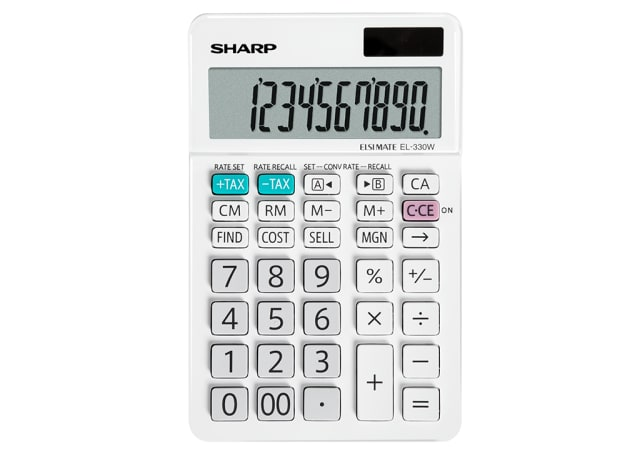EL330WB

# 10 Digit Angled Business Calculator - White

Desktop calculator with 10-digit display, Euro-conversion function.

POA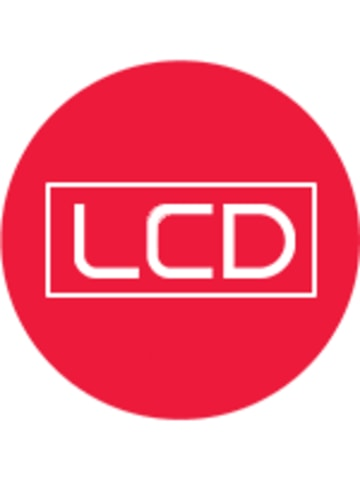## LCD Display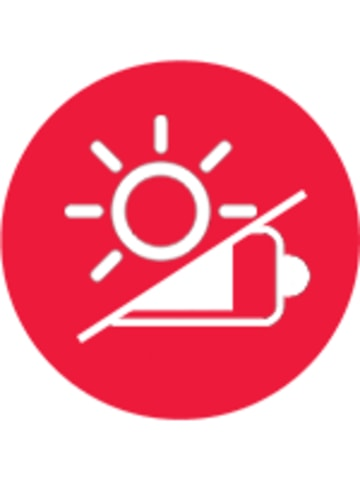## Twin Powered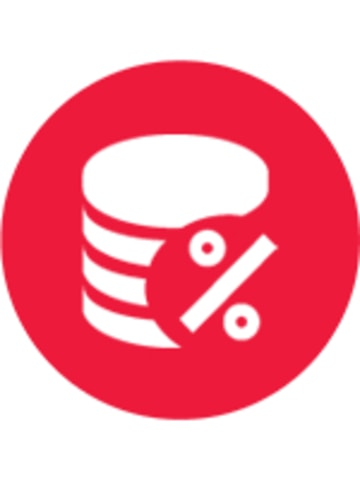## Cost Sell Margin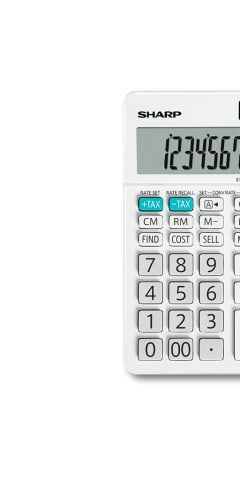• For easier reading of long, multi-digit numbers, "," or " , " is inserted for each group of three digits e.g. 10 thousand will be displayed as 10,000.
• To input two zeros by pressing the key once e.g. to input 100,  
• The calculator powers off automatically after approximately 7 minutes to save energy and prolong battery life.
• Change the result of calculation to positive or negative value.
• Recalls the value of the previous equation.
• To calculate certain percentage of a number e.g. 100 + 10% gives you 110. Great function for calculating before or after tax amount of a certain item.
• Determine the cost price for a certain margin, e.g. Cost price for 30% margin when the selling price is set at \$500 - input selling price [number] [SELL] input margin [number] [MGN] [FIND] [COST], number displayed will be the cost price. Great for small business owners to calculate and determine selling price.
Calculator Types
Display
10 Digit LCD display
Power Source
Solar / LR1130 x 1
Double Zero Key
Yes
Punctuation Digit
3
Yes
Memory
4
Cost Sell-Margin
Yes
Percentage
Yes
Sign Change
Yes
Auto Power Off
Yes